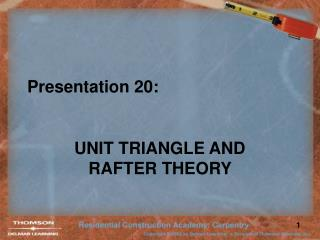DownloadDownload PresentationPresentation 20:

# Presentation 20:

Download Presentation## Presentation 20:

- - - - - - - - - - - - - - - - - - - - - - - - - - - E N D - - - - - - - - - - - - - - - - - - - - - - - - - - -
##### Presentation Transcript

1. Presentation 20: UNIT TRIANGLE AND RAFTER THEORY

2. Unit Triangle • Unit Triangle is found on the floor plans. • It may be in many shapes.

3. Unit Triangle vs. House • Doubling the Unit Triangle gives a shape that is similar to the building, but smaller.

4. Triangle Names • The sides of the triangles have names. Length Rise Run Length Rise Run Each side of the unit triangle has UNIT added to it.

5. Triangle Names • Thus the Unit Triangle Unit Length Unit Rise Unit Triangle Unit Run Building

6. 12′- 0″ Unit/House Relationship • How many units of run will fit into the building triangle? This building has six unit triangles. It depends on the size of the building.

7. Unit/House Relationship • How many units of rise will fit into the building triangle? In this building, six unit triangles.

8. Unit/House Relationship • How many units of length will fit into the building triangle? Again, six unit triangles.

9. 12′- 0″ Unit/House Relationship • There are equal numbers of unit triangles along the run, rise, and length. If this building is 12′ wide, then six unit triangles fit under the rafter.

10. Unit Triangle Theory • The base of the unit triangle is always 12″ for a common rafter. • The unit rise is given on the house plans. • If the unit rise is 6″, 13.42″ • Then the unit length is … 6″ Pythagorean Theorem 12″ = 1 foot = 1 unit of run or Rafter Tables

11. 20′- 0″ Example • If building is 20′ wide and unit rise is 6″, what is the rafter Total Rise and Line Length? 6″

12. 134 3/16″ 6″ 134 3/16″ 60″ 10′- 0″ = 10 units of run Rafter Calculation Answers • Total Rise = Unit Rise x RUN 6″ x 10 = 60″ • Line Length = Unit Length x RUN 13.42″ x 10 = 134.2″ = 134 3/16”

13. Conclusions 12″ • Unit run for a common rafter is ____. on the floor plans • The unit rise is found _____________. • Unit rise is converted to unit length by _______________________. using the Pythagorean Theorem Total Rise • Run x unit rise = _____________. Line Length • Run x unit length = _____________.